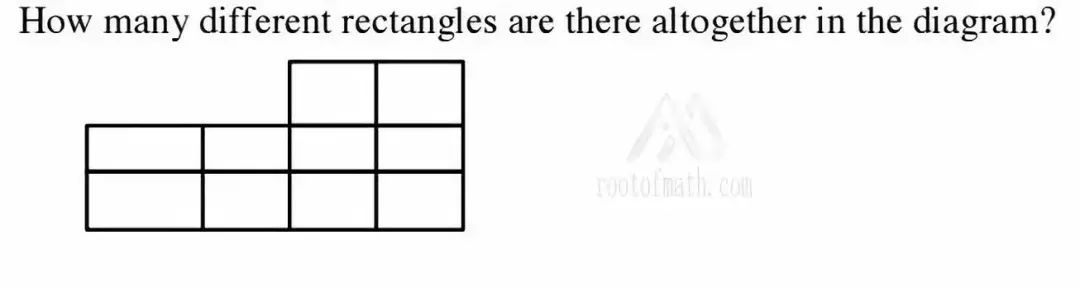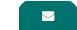## UBC Elmacon数学竞赛历年真题数据分析报告与备考

UBC&PIMS Elmacon号称加拿大难度最大的小学生数学竞赛”，对于激发孩子的数学学习兴趣和锻炼逻辑思维能力很有帮助，尤其是历届前十名的孩子很多进入了UBC天才班Transition Program进行深造学习，因此这一比赛越来越受到温哥华本地家长的关注和重视。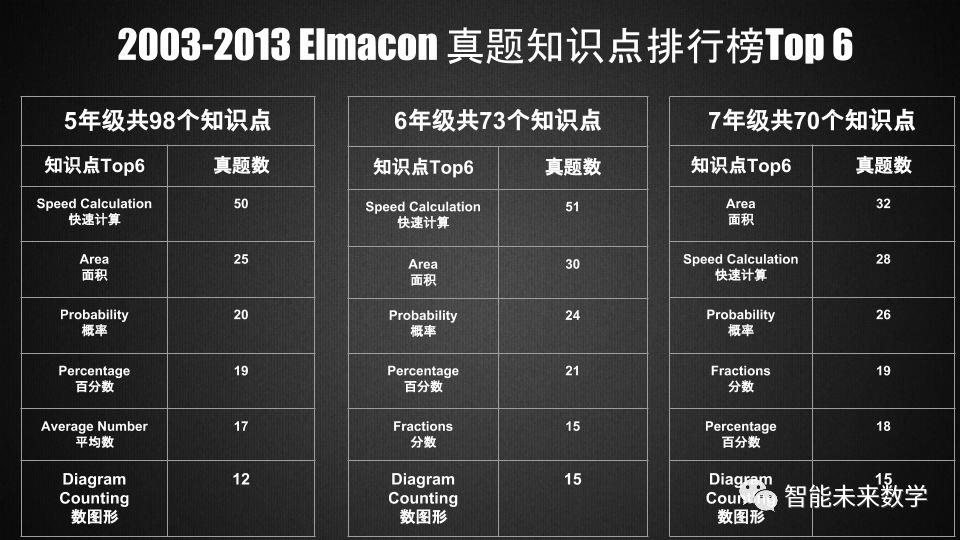Speed Calculation(快速计算)=6X(60-0.01)

=360-0.06

=359+1-0.06

=359.94Area(面积)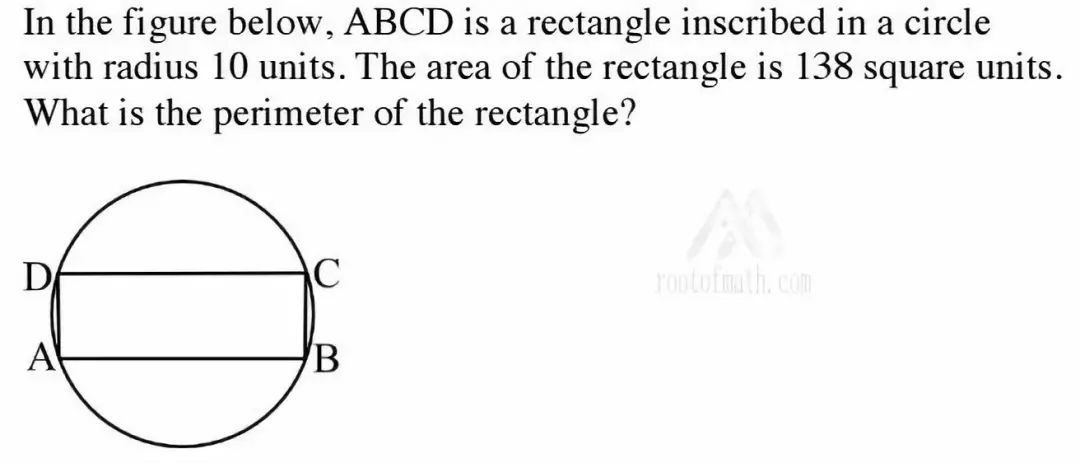Solution:假设长方形的边长为x,y,由题意可以得到：

(1) x*y=138

(2)x^2+y^2=20^2=400 (勾股定理)

(1) X 2+(2)左侧正好凑成完全平方公式：

(x+y)^2=2*x*y+x^2+y^2=2*138+400=576=26^2

Probability(概率)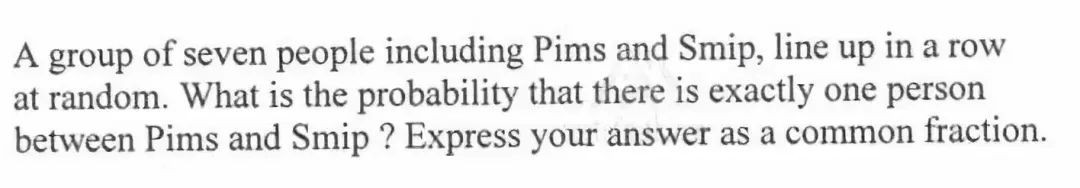Diagram Counting(数图形)